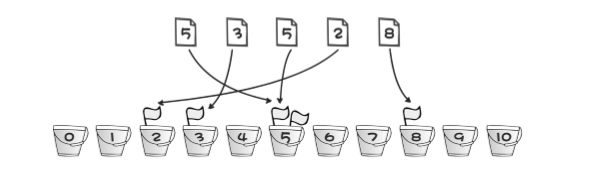### 一次刷题### 桶排• 要求排序的数在[1, n]区间。
• 开辟一个 n+1 大小的数组，每个元素就是一个空桶(a[n] = 0)。
• 将要排序的数依次标记到与数组下标相同的位置(如数 x，使 a[x]得到一次标记，比如 a[x]++)。
• 输出有标记的数值。

``````//bucketSort.cpp
#include<stdio.h>
int main()
{
int i, j, a, t;

for(i = 0; i < 10; i++)
a[i] = 0;

/* 输入并标记 */
for(i = 0; i < 5; i++)
{
scanf("%d", &t);
a[t]++;
}

/* 输出有标记的桶 */
for(i = 0; i < 10; i++)
for(j = 1; j <= a[i]; j++)
printf("%d ", i);

return 0;
}
``````

### 冒泡排序

5 2 8 6 7//第一次比较交换

5 8 2 6 7//第二次比较交换

5 8 6 2 7

5 8 6 7 2

``````//bubbleSort.cpp
#include<stdio.h>
int main()
{
int i, j, tmp, a = {2, 1, 4, 6, 5, 7, 9, 8, 0, 3};

for(i = 0; i < 9; i++) /* i只是为了标记还有多少个数需要冒泡 */
{
for(j = 0; j < 9 - i; j++) /* j每次从第一个相邻的数比较起，需要比较n-i-1次，因为后面的后面的数已经排列好了 */
{
if(a[j] > a[j+1]) /* 交换 */
{
tmp = a[j];
a[j] = a[j+1];
a[j+1] = tmp;
}
}
}

for(i = 0; i < 10; i++)
printf("%d ", a[i]);

return 0;
}
``````

//////未完待续